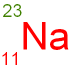gcsescience.com                                                 2                                                 gcsescience.com

Atomic Structure

What is the Difference between
the Atomic Number and the Mass Number?

What is the Atomic Number?

The number of protons in the nucleus of an atom
is called the atomic number.
Atoms of the same element have the same atomic number.

For sodium (Na) the atomic number is 11.
All sodium atoms must have 11 protons in their nucleus.
The atomic number therefore tells you what the element is
(see the periodic table).

What is the Mass Number?

The number of protons plus the number of neutrons
is called the mass number.
Sodium has 11 protons and 12 neutrons.
The mass number is 11 + 12  =  23.

The mass number is the total number of particles in the nucleus.
The mass number and the atomic number are written
above and below the chemical symbol for the element, as shown.What is an Isotope?

Atoms of the same element may have different numbers
of neutrons. These are called isotopes.
For example, Chlorine (atomic number 17)
may have 18 or 20 neutrons.
The mass number will be
either 17 + 18  =  35, or 17 + 20  =  37.

Isotopes of the same element have the
same chemical properties because the number of
protons and electrons are the same.